High School Physics : Electricity

Example Questions

← Previous 1

Example Question #1 : Electricity And Magnetism

Calculate the magnitude of the electric field at a point that is located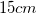directly north of a point charge,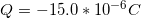.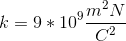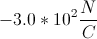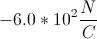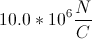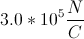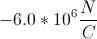Explanation:

The magnitude of an electric field due to a single point charge is given by the formula: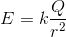The variablerefers to Coulomb's constant. We are given the charge and the distance between points. To use the equation, first convert the distance to meters.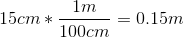We can now use the given constant, charge, and distance in the equation to solve for the magnetic field strength.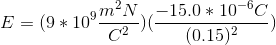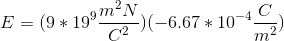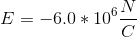Example Question #1 : Electric Charge

Materials in which the electrons are bound very loosely to the nuclei and can move about freely within the material are referred to as

Semiconductors

Insulators

Conductors

Superconductors

Conductors

Explanation:

Conductors allow the electrons to flow freely along it.  That is why metal; is considered a good conductor.  It allows the electrons to flow through it which is why it is used in wire in an an electric circuit.

Example Question #2 : Electric Charge

A glass rod is rubbed with a piece of silk.  During the process the glass rod acquires a positive charge.  The silk

Could either be positively charged or negatively charged.  It depends on how hard the rod was rubbed.

Remains neutral

Acquires a positive charge as well

Acquires a negative charge

Acquires a negative charge

Explanation:

Since the glass rod acquires a positive charge, this means that it is deficient in electrons. Since the rod was rubbed by the piece of silk, the silk is what now collects the electrons.  The silk now has an excess of electrons which means that the silk is now negatively charged.

Example Question #3 : Electric Charge

A charged rod carrying a negative charge is brought near two spheres that are in contact with each other but insulated from the ground. If the two spheres are then separated, what kind of charge will be on the spheres?

The sphere near the charged rod becomes negative and the other becomes positive

The sphere near the charged rod becomes positive and the other becomes negative

The spheres do not get any charge

None of these

The sphere near the charged rod becomes positive and the other becomes negative

Explanation:

When the negatively charged rod is brought near one of the two spheres, the presents of the negative charge will induce a flow of charge in the spheres such that regions farthest away from the charged rod will become most negative and regions near the rod will become most positive. This is called charge by induction.

Example Question #4 : Electric Charge

By what method will a positively charged rod produce a negative charge on a conducting sphere that is placed on an insulating surface?

Charge by conduction

None of these

Charge by convection

Charge by induction

Charge by induction

Explanation:

Charge by induction happens when a charged object is brought in the vicinity of a neutral object. The presents of the charged object will cause the free charges in the neutral object to shift such that the neutral object becomes polarized. When the charged object is positive, this will induce a negative charge on a neutral object.

Example Question #5 : Electric Charge

How many electrons make up a charge of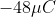?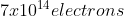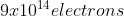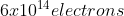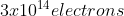Explanation:

The charge of a single electron is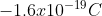We can then convert the amount of charge to determine the number of electrons.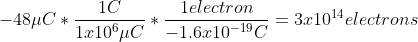Example Question #6 : Electric Charge

A neutral atom always has

More neutrons than protons

More protons than electrons

The same number of neutrons and protons

The same number of protons and electrons

The same number of protons and electrons

Explanation:

Protons are positively charged and electrons are negatively charged.  In order for an object to be considered neutral, it must have the same number of both positive and negative particles.  Therefore it must have the same number of both protons and electrons.

Example Question #7 : Electric Charge

As an object acquires a positive charge, its mass usually

Becomes negative

Increases

Stays the same

Decreases

Decreases

Explanation:

When an object acquires a positive charge, it is losing electrons to its surroundings.  If it is losing electrons, it is losing mass (although ever so slightly).

Example Question #8 : Electric Charge

What is the net charge of abar of gold?  Gold has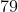electrons per atom and an atomic mass of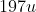.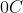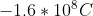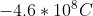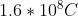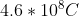Explanation:

Since a gold atom has balanced protons and electrons, the net charge on the atom is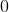.

Example Question #9 : Electric Charge

A negative point charge is in an electric field created by a positive point charge. Which of the following is true?

The field points away from the positive charge, and the force on the negative charge is in the same direction as the field

The field points toward the positive charge and the force on the negative charge is in the opposite direction as the field

The field points toward the positive charge and the force on the negative charge is in the same direction as the field

The field points away from the positive charge, and the force on the negative charge is in the opposite direction as the field